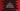# C fsetpos method explanation with example## C fsetpos method explanation with example:

fsetpos method is used to set the file position indicator for a given file stream based on a given value.

In this post, we will learn the definition of fsetpos and how to use it with examples.

## Definition of fsetpos:

fsetpos is defined in the header stdio.h and it is defined as like below:

``int fsetpos( FILE *stream, const fpos_t *pos );``

As I have mentioned above, this method is used to set the file position indicator for a given file stream, which is stream in the above definition. pos is the new value of file position indicator. It is a pointer to a fpos_t object.

### Return value of fsetpos:

It returns a zero for success and any other value for failed.

To get the fpos_t value, we will use the fgetpos method.

## Example of fsetpos:

Let’s take an example of fsetpos and how to use it:

``````#include <stdio.h>

int main()
{
FILE *f;
fpos_t p;

fputs("Hello", f);

fgetpos(f, &p);

fputs("World", f);

fsetpos(f, &p);

fputs("Universe", f);

fclose(f);

return 0;
}``````

In this program,

• f is a FILE pointer and p is a fpos_t variable.
• We are opening one file readme.md in w mode.
• It first writes Hello to the file. Then it gets the position of the file position indicator and puts that in the p variable.
• It writes World after that to the file.
• Then, we are calling fsetpos to move the indicator to the previous position, i.e. it will move it to the position before World was written.
• It is writing Universe. But since we have used fsetpos to move the indicator, it will write Universe after Hello and replace World.

If you open the file, it will hold the below text:

``HelloUniverse``

### Check the return value of fsetpos:

We can also check the return value of fsetpos. fsetpost returns 0 for success, so we can rewrite the above program as like below:

``````#include <stdio.h>

int main()
{
FILE *f;
fpos_t p;

fputs("Hello", f);

if (fgetpos(f, &p) == 0)
{
fputs("World", f);

fsetpos(f, &p);

fputs("Universe", f);
}

fclose(f);

return 0;
}``````

i.e. we are doing other operation only if fgetpos returns 0 or only if the call of fgetpos is success.

If you run the above code, it will give similar output.